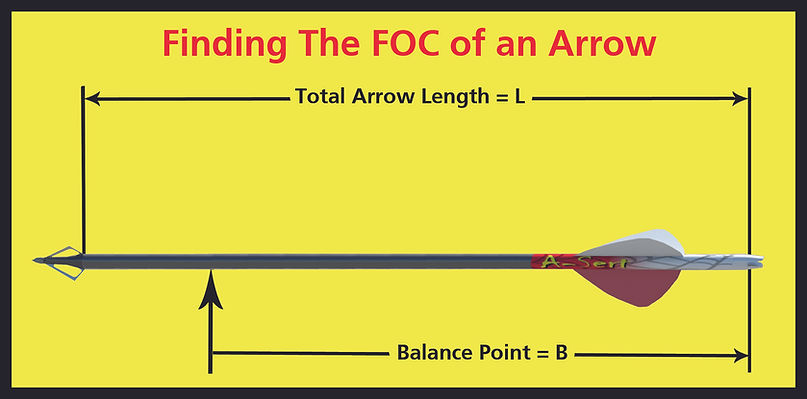How to Calculate Front of Center (FOC)

B=Balance Point - measured from throat of nock to the point of balance

L=Arrow Length - measured from throat of nock to the end of arrow shaft

1. Measure the arrow length from the throat of the nock to the end of the shaft.

(e.g.  28.5)

2. Divide the total arrow length by two (2). Or multiply arrow length by 0.5.

(e.g. 28.5" / 2" = 14.25" or 28.5" x 0.5" = 14.25")

3. Find the arrow's balance point. Measure from the nock throat to the balance point.

(e.g. 19.0")

4. Subtract half the total length from the balance point number.

(e.g. 19.0" - 14.25" = 4.75")

5. Multiply that result by 100.

(e.g. 4.75" x 100 = 475)

6. Now divide that number by the total arrow length.

(e.g. 475 / 28.5 = 16.6% The FOC measurement is 16.6%)

The AMO-Standard FOC Balance Formula is: %FOC = (100x(B-L/2))/L